# Civil Engineering - Theory of Structures

### Exercise :: Theory of Structures - Section 3

21.

The equivalent length is of a column of length L having both the ends fixed, is

 A. 2L B. L C.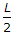D.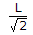Explanation:

No answer description available for this question. Let us discuss.

22.

The moment of inertia of a rectangular section of width B and depth D about an axis passing through C.G. and parallel to its width is

 A.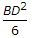B.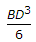C.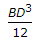D.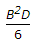E.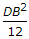Explanation:

No answer description available for this question. Let us discuss.

23.

A masonry dam (density = 20, 000 N/m3) 6 m high, one metre wide at the top and 4 m wide at the base, has vertical water face. The minimum stress at the base of the dam when the reservoir is full, will be

 A. 75 N/m2 B. 750 N/m2 C. 7500 N/m2 D. 75000 N/m2

Explanation:

No answer description available for this question. Let us discuss.

24.

The ratio of tangential and normal components of a stress on an inclined plane through θ° to the direction of the force, is :

 A. sin θ B. cos θ C. tan θ D. cos θ E. sec θ

Explanation:

No answer description available for this question. Let us discuss.

25.

For calculating the allowable stress of long columns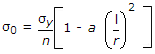is the empirical formula, known as

 A. Straight line formula B. Parabolic formula C. Perry's formula D. Rankine's formula.